Sports analytics are becoming more popular and various advanced stats are commonly used not only within sports organizations, but in the media as well. They are probably most popular in the NBA, but the NFL has a few worthwhile advanced stats. This article by Michael Renner uses a stat called called Expected Points Added, which is fully explained here. Basically this is a better measure of the value of each play taking into account down, distance, and field positioning.

Taysom Hill doing Taysom Hill ???? @T_Hill4 pic.twitter.com/CDmQpt5hlE

— The Checkdown (@thecheckdown) November 4, 2018

Renner does a great job showing why Taysom Hill is so valuable. I decided to take a deeper look at EPA and compare Taysom Hill to Lamar Jackson, who has often been utilized similar to Taysom Hill this season. For those who like to do their own analysis, I have included the R code I used to do the analysis. I make use of a handy package called “nflscrapR”, which basically allows you to get detailed play by play information and manipulate it using R. R is an open source and free programming language that is primarily geared towards statistical analysis and is popular for both academic and commercial use.

Data

library(tidyverse)
library(nflscrapR)
library(knitr)
# remove the '#' to run. I have it commented out to avoid slow compiling time, as it takes a while to download all of the data.
# pbp_2018 <- season_play_by_play(2018)

Then I get the specific stats for both players for every time they made a play as a quarterback, running back, or receiver.

pid <- c("00-0033357","00-0034796")
df <- bind_rows(pbp_2018 %>% filter(Passer_ID %in% pid),
pbp_2018 %>% filter(Rusher_ID %in% pid),
x <- df %>% filter(Passer_ID == pid | Rusher_ID == pid | Receiver_ID == pid) %>% mutate(Player = "T.Hill")
y <- df %>% filter(Passer_ID == pid | Rusher_ID == pid | Receiver_ID == pid) %>% mutate(Player = "L.Jackson")
df <- bind_rows(x,y)

First, let’s look at a more traditional measure of success. Taysom Hill is often used in short yardage situations where a first down is the primary goal. I have watched fewer Baltimore games, but I assume Lamar is often used in the same situations. Regardless, this data shows how often they get a first down when they are involved in a play.

all <- pbp_2018 %>% filter(PlayType %in% c('Pass','Run'))
x <- df %>% group_by(Player) %>% summarise_at(vars(FirstDown), sum)
y <- df %>% count(Player)
x <- left_join(x,y,by = "Player")
x <- x %>% mutate(pct = FirstDown/n*100,
NFLaverage = (sum(all\$FirstDown, na.rm = T) / nrow(all))*100,
difference = pct - NFLaverage)
names(x) <- c("Player","First Downs","Total Plays",'First Down %',"NFL Average","Difference")
kable(x, digits = 2)
Player First Downs Total Plays First Down % NFL Average Difference
L.Jackson 12 43 27.91 27.56 0.35
T.Hill 13 35 37.14 27.56 9.58

Taysom Hill is better than the NFL average by almost 10%, while Jackson is just a little above the NFL average.

Now lets take a look at the advanced stat EPA along with average yards gained, which is a more traditional and intuitive metric.

kable(df %>% group_by(Player) %>% summarise_at(vars(EPA, Yards.Gained), mean, na.rm = T), digits = 2)
Player EPA Yards.Gained
L.Jackson 0.06 5.07
T.Hill 0.38 5.91
plotTitle <- "EPA comparison"

ggplot(df, aes(x = Player, y = EPA, fill = Player)) + geom_boxplot() +
guides(fill = F) + theme_bw()  +
ggtitle(plotTitle)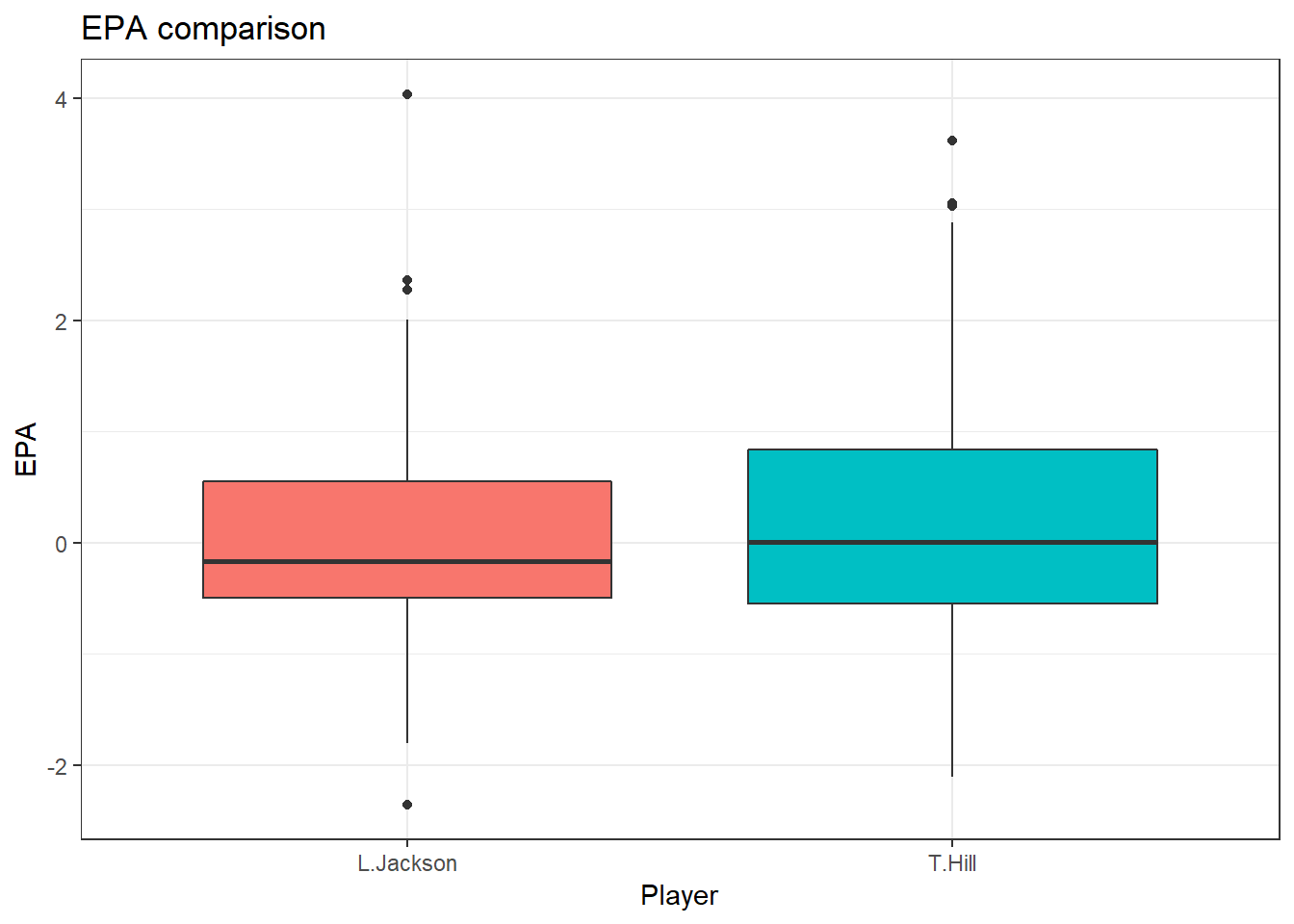plotTitle <- "Yards gained comparison"

ggplot(df, aes(x = Player, y = Yards.Gained, fill = Player)) + geom_boxplot() +
guides(fill = F) + theme_bw()  +
ggtitle(plotTitle)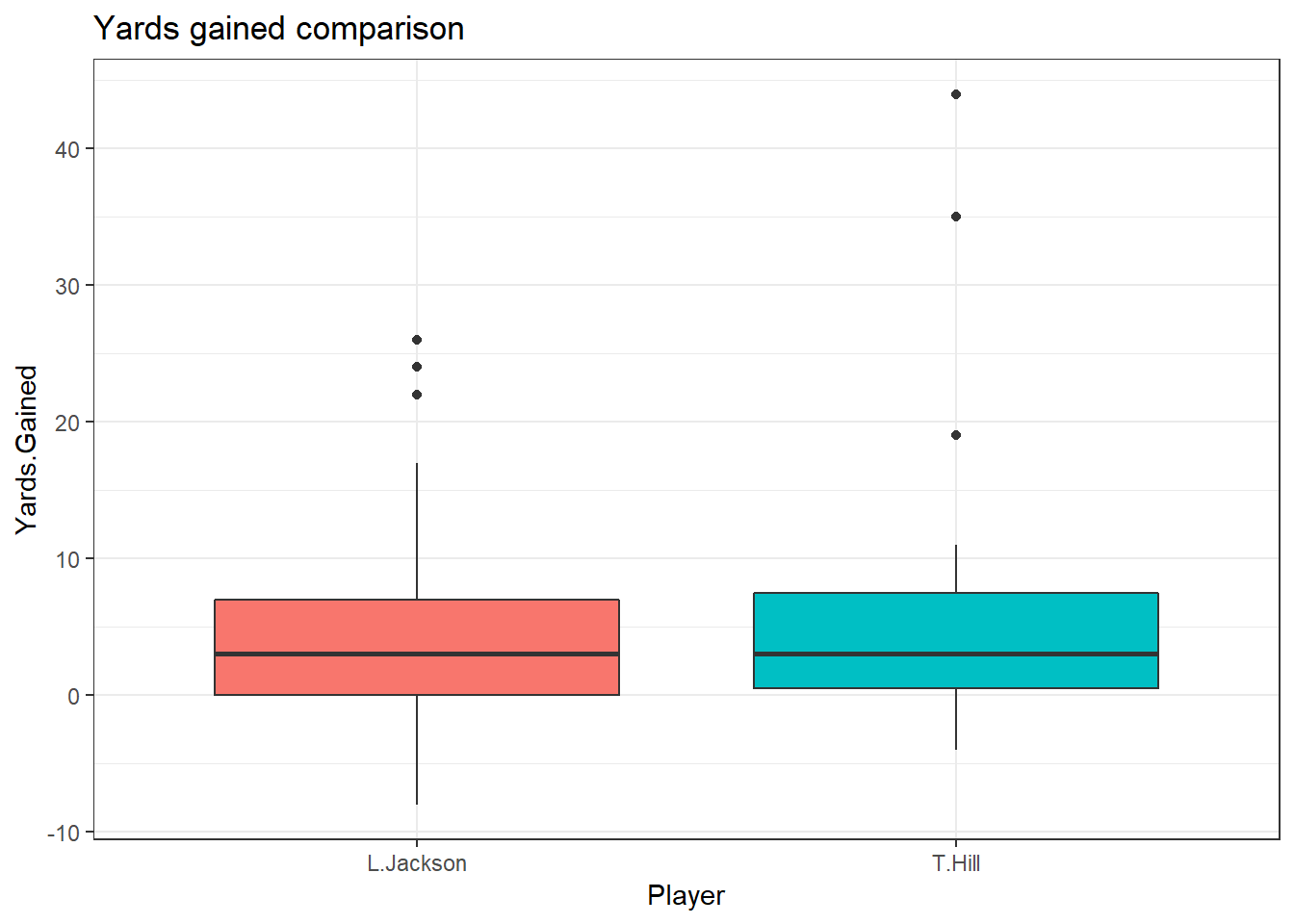Taysom Hill’s EPA is much higher than Lamar Jackson’s, but his average yards gained is less than a yard better. This shows that Taysom Hill’s plays are generally more impactful, while not gaining a ton more yards.

The EPA and yards gained stats are hard to gauge without comparisons. Here are the NFL averages for EPA and yards gained (average).

allTeam <- all %>% group_by(posteam) %>% summarise_at(vars(EPA,Yards.Gained), mean, na.rm = T)
allTeam <- allTeam[-1,] # get rid of NA
plotdf <- df %>% select(Player,EPA,Yards.Gained)
plotdf <- plotdf %>% group_by(Player) %>% summarise_all(mean)
names(plotdf) <- "posteam"
plotdf <- bind_rows(plotdf,allTeam)

plotTitle <- "Average EPA"

ggplot(plotdf, aes(EPA,reorder(posteam,EPA), color = posteam)) + geom_point() + theme_bw() +
guides(color = F) + ylab("") +
geom_vline(xintercept = desc <- as.vector(summary(allTeam\$EPA))[c(2,4:5)], lwd = 2,
color = "red", alpha = .5) +
geom_point(data = plotdf[1:2,], aes(EPA,posteam), size = 4, color = "Purple") +
ggtitle(plotTitle)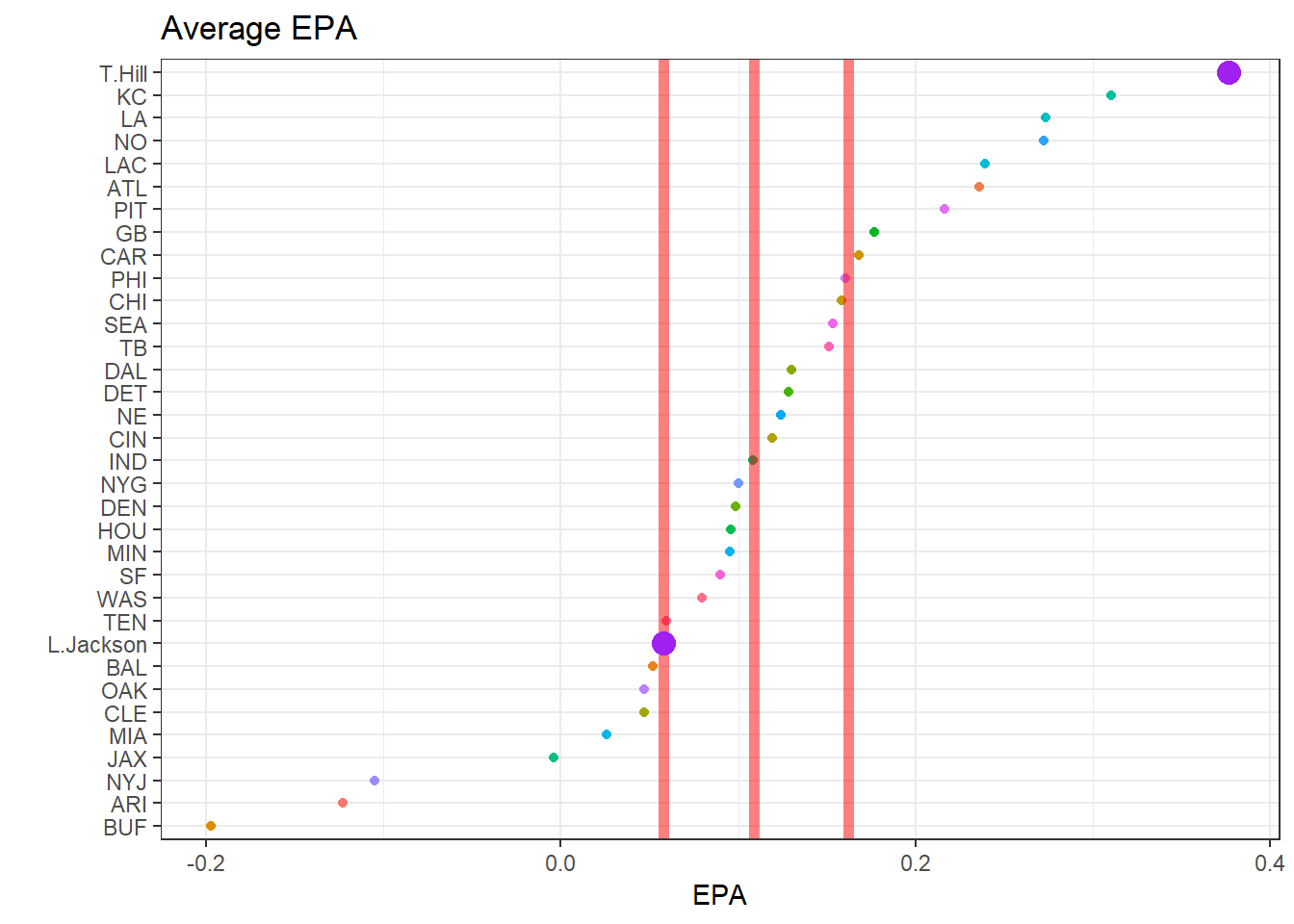plotTitle <- "Average yards gained"

ggplot(plotdf, aes(Yards.Gained,reorder(posteam,Yards.Gained), color = posteam)) + geom_point() + theme_bw() +
guides(color = F) + ylab("") +
geom_vline(xintercept = desc <- as.vector(summary(allTeam\$Yards.Gained))[c(2,4:5)], lwd = 2,
color = "red", alpha = .5) +
geom_point(data = plotdf[1:2,], aes(Yards.Gained,posteam), size = 4, color = "Purple") +
ggtitle(plotTitle)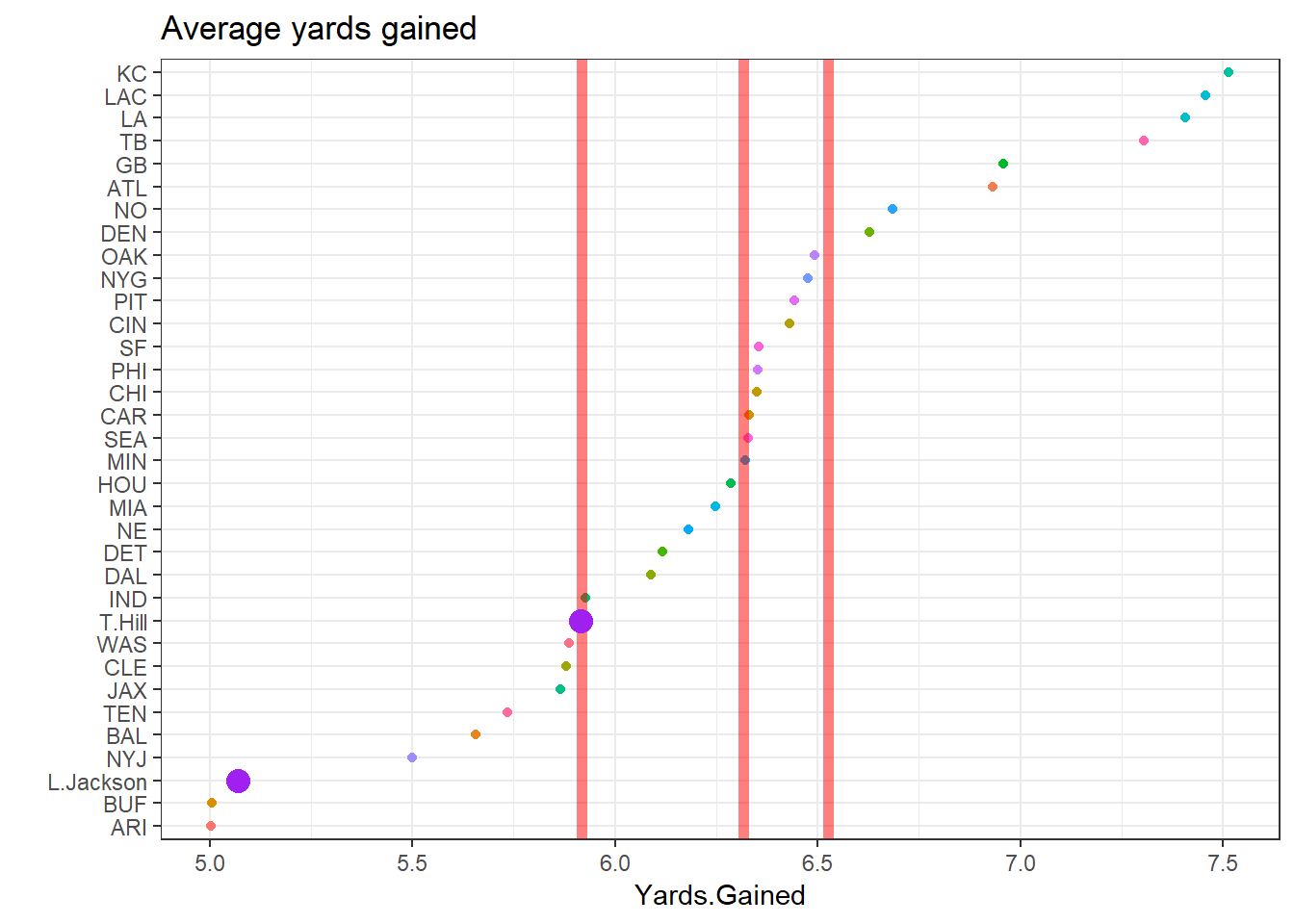Note that the three bars represent the first quartile, mean, and third quartile for all plots where included.

For EPA, Hill is above average for every team, while Jackson is right on the first quartile. Yards gained shows a much different story. This is mainly an effect of the passing game, but also shows why EPA is much more valuable at showing how each player impacts the game.

Now lets take a look at how they compare to the top twenty running backs by total yards gained.

df1 <- pbp_2018 %>% group_by(Rusher) %>% summarise_at(vars(Yards.Gained, EPA),sum)
df1 <- df1 %>% filter(!is.na(Rusher))  %>% arrange(desc(Yards.Gained))
df1 <- df1 %>% top_n(20,Yards.Gained)
df1 <- all %>% filter(Rusher %in% df1\$Rusher)
df1 <- df1 %>% group_by(Rusher) %>% summarise_at(vars(EPA,Yards.Gained),mean)
plotdf <- df %>% select(Player,EPA,Yards.Gained)
plotdf <- plotdf %>% group_by(Player) %>% summarise_all(mean)
names(plotdf) <- "Rusher"
plotdf <- bind_rows(plotdf,df1)

plotTitle <- "Average EPA compared to top 20 RBs"

ggplot(plotdf, aes(EPA,reorder(Rusher,EPA), color = Rusher)) + geom_point() + theme_bw() +
guides(color = F) + ylab("") +
geom_vline(xintercept = desc <- as.vector(summary(df1\$EPA))[c(2,4:5)], lwd = 2,
color = "red", alpha = .5) +
geom_point(data = plotdf[1:2,], aes(EPA,Rusher), size = 4, color = "Purple") +
ggtitle(plotTitle)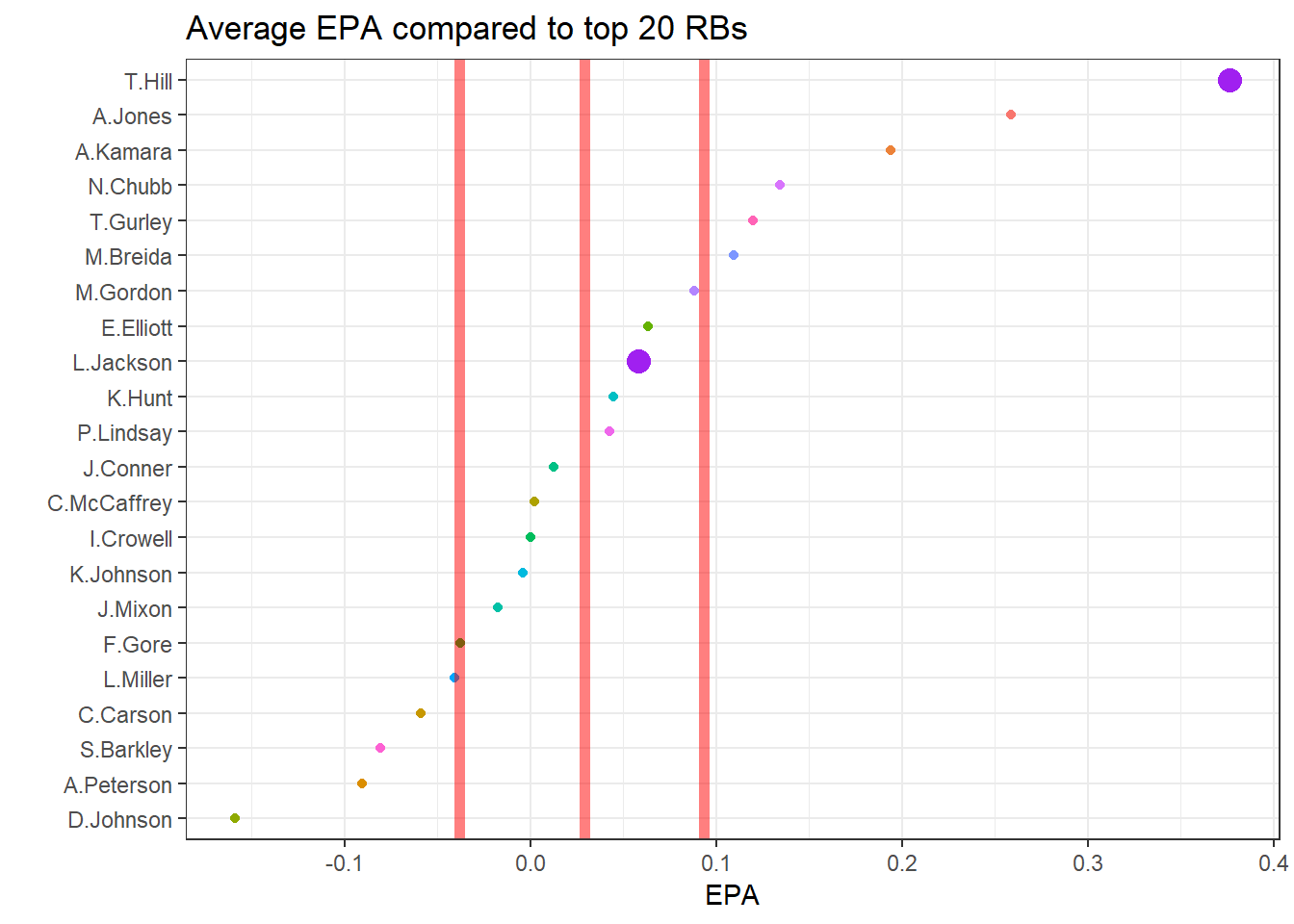plotTitle <- "Average yards gained compared to top 20 RBs"

ggplot(plotdf, aes(Yards.Gained,reorder(Rusher,Yards.Gained), color = Rusher)) + geom_point() + theme_bw() +
guides(color = F) + ylab("") +
geom_vline(xintercept = desc <- as.vector(summary(df1\$Yards.Gained))[c(2,4:5)], lwd = 2,
color = "red", alpha = .5) +
geom_point(data = plotdf[1:2,], aes(Yards.Gained,Rusher), size = 4, color = "Purple") +
ggtitle(plotTitle)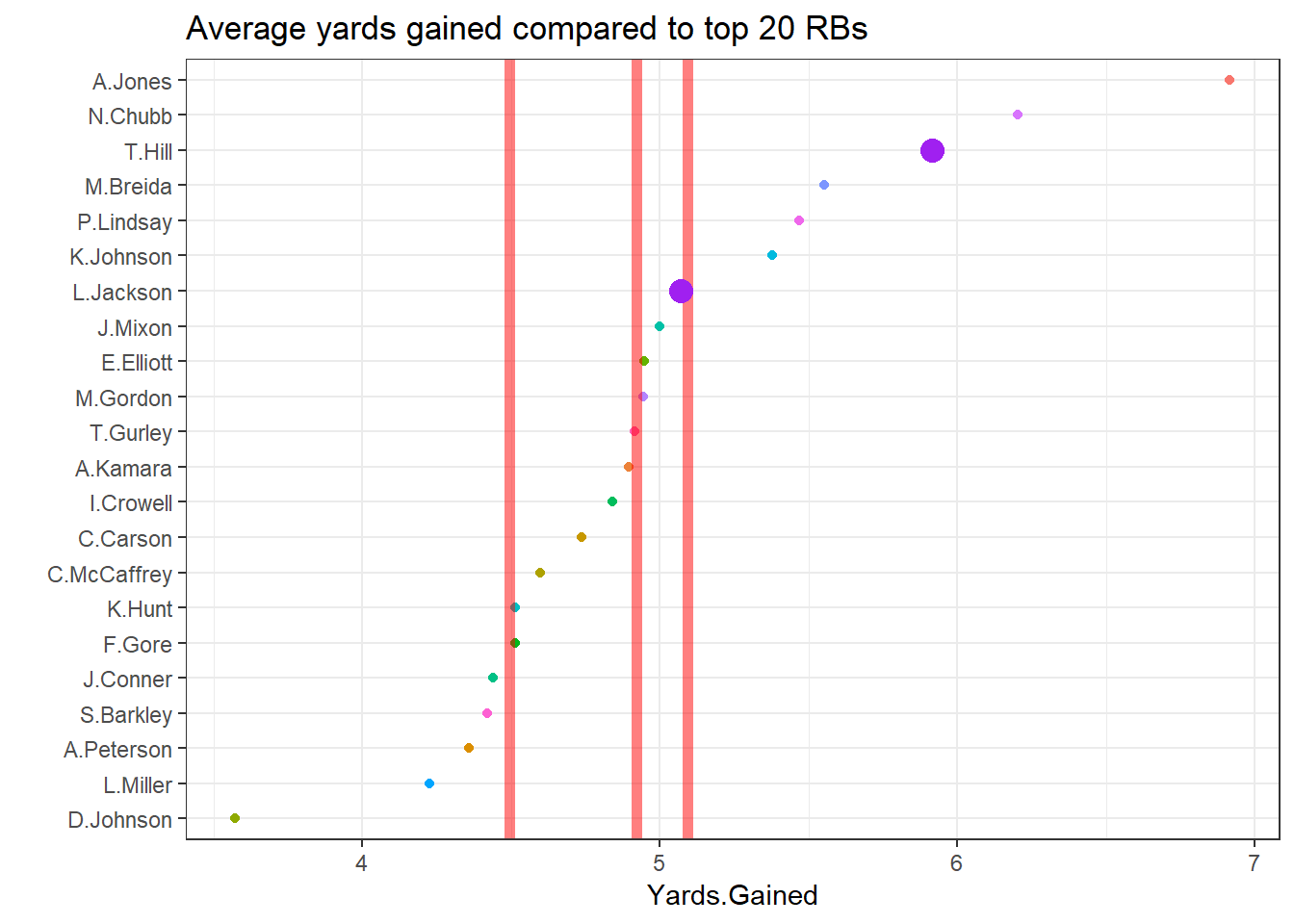Both Hill and Jackson stack up favorably compared to these running backs. Hill has a considerably higher EPA than the next closest running back as well as his teammate Alvin Kamara.

Next lets compare them to the top quarterbacks.

df1 <- pbp_2018 %>% group_by(Passer) %>% summarise_at(vars(Yards.Gained, EPA),sum)
df1 <- df1 %>% filter(!is.na(Passer),!is.na(EPA))  %>% arrange(desc(Yards.Gained))
df1 <- df1 %>% top_n(30,Yards.Gained)
df1 <- all %>% filter(Passer %in% df1\$Passer)
df1 <- df1 %>% group_by(Passer) %>% summarise_at(vars(EPA,Yards.Gained),mean)
plotdf <- df %>% select(Player,EPA,Yards.Gained)
plotdf <- plotdf %>% group_by(Player) %>% summarise_all(mean)
names(plotdf) <- "Passer"
plotdf <- bind_rows(plotdf,df1)

plotTitle <- "Average EPA compared to top 20 QBs"

ggplot(plotdf, aes(EPA,reorder(Passer,EPA), color = Passer)) + geom_point() + theme_bw() +
guides(color = F) + ylab("") +
geom_vline(xintercept = desc <- as.vector(summary(df1\$EPA))[c(2,4:5)], lwd = 2,
color = "red", alpha = .5) +
geom_point(data = plotdf[1:2,], aes(EPA,Passer), size = 4, color = "Purple") +
ggtitle(plotTitle)